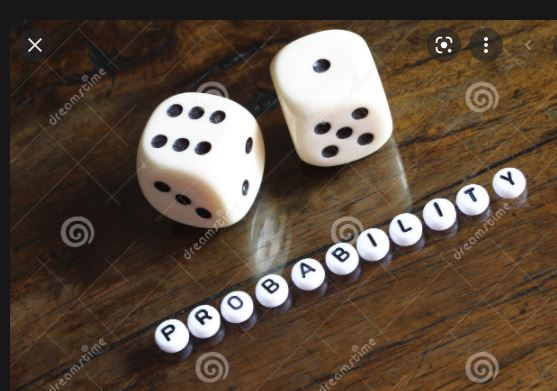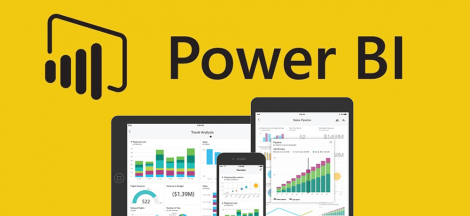# DATA ANALYTICS – INTRODUCTION TO PROBABILITY BY DR. BONGO ADI. – PART 1The understanding of the topic coming from my university days seem to look very difficult and a bit confusing for me. Most time I approach the topic with a gabbage-in, gabbage-out approach without understanding the principles behind most of the calculations. I used to be concerned about the formulae without considering the applications of the calculations to real life situations. All that matters is cramming the formulars and download to solve statistical problems like the probability’s questions during the exam period. I virtually study for exam and not for application. This idea was totally discouraged as I encountered Dr. Bongo Adi, a well learned facilitators who understands students’ psychology to learning for examination purposes. Dr. changed my orientation as he approached the topic with intelligence and dexterity; keeping my focus on the application of the topic rather than learning to pour during the examination time. He did not outrightly condemn learning to pass but emphasized the need to understand the topic for application as managers who are meant to make business decisions and also make recommendations to the management whenever the need arises.

I honestly appreciate Dr. Bongo from the way he introduced the topic.  He started by looking at the following:

Objectives of Probabilities

1. To obtain an understanding of the role probability information plays in the decision-making process.
2. Understand probability as a numerical measure of the likelihood of occurrence.
3. Be able to use the three methods (classical, relative frequency, and subjective) commonly used for assigning probabilities and understand when they should be used.
4. Be able to use the addition law and be able to compute the probabilities of events using conditional probability and the multiplication law.
5. Be able to use new information to revise initial (prior) probability estimates using Bayes’ theorem.

Terminologies.

He also looked at various terms in probabilities such as:

• An experiment is any process that generates well defined outcomes.
• The sample space for an experiment is the set of all experimental outcomes.
• An experimental outcome is also called a sample point.
• An event is a collection of sample points. The probability of any event is equal to the sum of the probabilities of the sample points in the event. If we can identify all the sample points of an experiment and assign a probability to each, we can compute the probability of an event

Assigning Probabilities:

1. The probability assigned to each experimental outcome must be between 0 and 1, inclusively.
2. The sum of the probabilities for all experimental outcomes must equal 1.

Probability Methods:

1. Classical Method – Assigning probabilities based on the assumption of equally likely outcomes. e.g rolling of a die
2. Relative Frequency Method – Assigning probabilities based on experimentation or historical data
3. Subjective Method – Assigning probabilities based on judgment

We shall stop at the probability methods and continue with some relationships with probabilities – Addition Law, Complements, Multiplication Laws and conditional probabilities law.

#EMBA27## Unveiling the Power of Data Visualization with Power BI

in## The Essence of Brand and Personal Branding

in